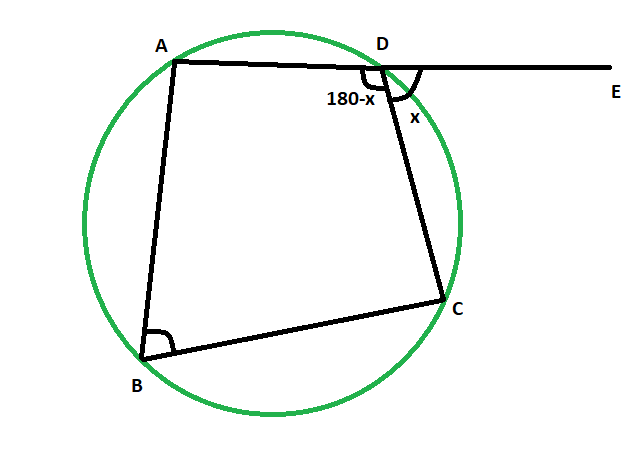# Exterior angle of a cyclic quadrilateral when the opposite interior angle is given

• Last Updated : 22 Mar, 2021

Given cyclic quadrilateral inside a circle, the task is to find the exterior angle of the cyclic quadrilateral when the opposite interior angle is given.
Examples:

```Input: 48
Output: 48 degrees

Input: 83
Output: 83 degrees```Approach

• Let, the exterior angle, angle CDE = x
• and, it’s opposite interior angle is angle ABC
• as, ADE is a straight line
• so, angle ADC = (180-x) degrees
• since, opposite angles of a cyclic quadrilateral are supplementary,
• angle ABC = xBelow is the implementation of the above approach:

## C++

 `// C++ program to find the exterior angle``// of a cyclic quadrilateral when``// the opposite interior angle is given` `#include ``using` `namespace` `std;` `void` `angleextcycquad(``int` `z)``{``    ``cout << ``"The exterior angle of the"``         ``<< ``" cyclic quadrilateral is "``         ``<< z << ``" degrees"` `<< endl;``}` `// Driver code``int` `main()``{``    ``int` `z = 48;``    ``angleextcycquad(z);``    ``return` `0;``}`

## Java

 `// Java program to find the exterior angle``// of a cyclic quadrilateral when``// the opposite interior angle is given` `import` `java.io.*;` `class` `GFG``{` `static` `void` `angleextcycquad(``int` `z)``{``    ``System.out.print( ``"The exterior angle of the"``        ``+ ``" cyclic quadrilateral is "``        ``+ z +``" degrees"``);``}` `// Driver code``public` `static` `void` `main (String[] args)``{``        ``int` `z = ``48``;``    ``angleextcycquad(z);``}``}` `// This code is contributed by anuj_67..`

## Python3

 `# Python program to find the exterior angle``# of a cyclic quadrilateral when``# the opposite interior angle is given` `def` `angleextcycquad(z):``    ``print``(``"The exterior angle of the"``,end``=``"");``    ``print``(``"cyclic quadrilateral is "``,end``=``"");``    ``print``(z,``" degrees"``);` `# Driver code``z ``=` `48``;``angleextcycquad(z);` `# This code is contributed by 29AjayKumar`

## C#

 `// C# program to find the exterior angle``// of a cyclic quadrilateral when``// the opposite interior angle is given``using` `System;` `class` `GFG``{` `static` `void` `angleextcycquad(``int` `z)``{``    ``Console.WriteLine( ``"The exterior angle of the"``        ``+ ``" cyclic quadrilateral is "``        ``+ z +``" degrees"``);``}` `// Driver code``public` `static` `void` `Main ()``{``        ``int` `z = 48;``    ``angleextcycquad(z);``}``}` `// This code is contributed by anuj_67..`

## Javascript

 ``
Output:
`The exterior angle of the cyclic quadrilateral is 48 degrees`

My Personal Notes arrow_drop_up